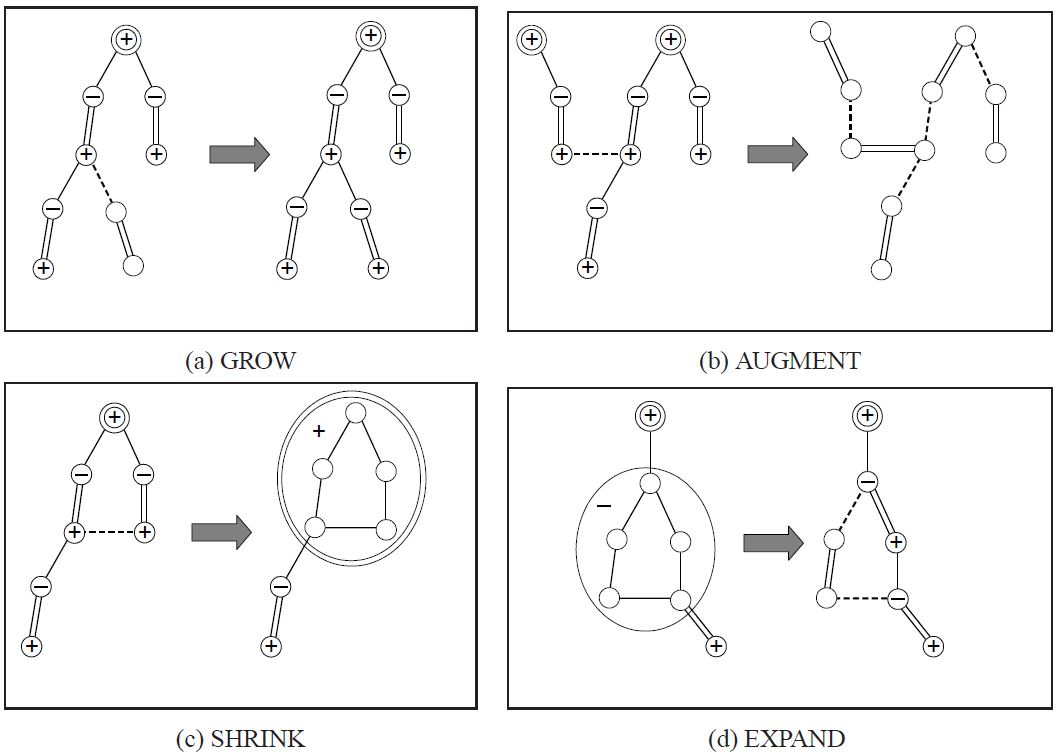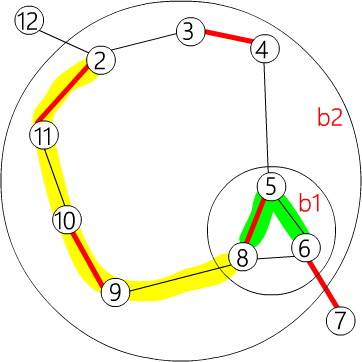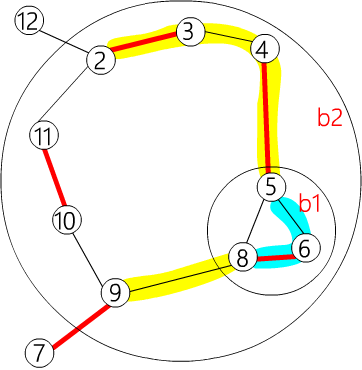# 一般图最大权匹配

## 一般图最大权完美匹配的线性规划

### 定义### 对偶问题的边是匹配边，的边是非匹配边。和二分图一样，我们必须满足。因此必须在最大权完美匹配的时候，让所有匹配边都是 等边 的。

• 对于选中的边，必有• 对于选中的集合B，即所有的集合，都被选了集合大小一半的边，也即集合是一朵花，选中花中的一条边进行增广。同时，我们加入一个条件：，即只有花向外连了一条边的时候，才是有意义的。### 算法的四个步骤

1. GROW（等边）：用 "等边" 构成交错树。
2. AUGMENT（增广）：找出增广路并扩充匹配。
3. SHRINK（缩花）：把花缩成一个点。
4. EXPAND（展开）：把花拆开。### 调整 VERTEX LABELING

vertex labeling 仍要维持大于等于的性质，而且既有的「等边」不能被改变，还要让尽量的小。来表示在交错树上为奇点。来表示在交错树上为偶点。来表示不在任何一棵交错树上。，让## 一般图最大权匹配

vertex labeling 为的点最后将成为未匹配点。

### 参考代码

  1 2 3 4 5 6 7 8 9 10 11 12 13 14 15 16 17 18 19 20 #define INF INT_MAX #define MAXN 400 struct edge { int u, v, w; // 表示(u,v)为一条边其权重为w edge() {} edge(int u, int v, int w) : u(u), v(v), w(w) {} }; int n, n_x; // 有n个点，编号为 1 ~ n // n_x表示当前点加上花的数量，编号从n+1到n_x为花的节点 edge g[MAXN * 2 + 1][MAXN * 2 + 1]; // 图用邻接矩阵存储，因为最多有n-1朵花，所以大小为MAXN* vector flower[MAXN * 2 + 1]; // flower[b]记录了花b中有哪些点 // 我们记录花中的点的方式是只记录花里面的最外层花1 2 flower[b2] = {b1, 4, 3, 2, 11, 10, 9} flower[b1] = {6, 5, 8}1 2 flower[b2] = {9, b1, 4, 3, 2, 11, 10} flower[b1] = {5, 8, 6} 
  1 2 3 4 5 6 7 8 9 10 11 12 13 14 15 16 17 int lab[MAXN * 2 + 1]; // lab[u]用来记录z_u, lab[b]用来记录z_B int match[MAXN * 2 + 1], slack[MAXN * 2 + 1], st[MAXN * 2 + 1], pa[MAXN * 2 + 1]; // match[x]=y表示(x,y)是匹配，这里x、y可能是花 // slack[x]=u表示z(x,u)是所有和x相邻的边中最小的那条边 // 表示节点 x 所在的花是 b。如果 x=b 且 b<=n，则表示 x // 是一个普通节点（不属于任何花） 表示在交错树中，节点 v 的父节点是 u int flower_from[MAXN * 2 + 1][MAXN + 1], S[MAXN * 2 + 1], vis[MAXN * 2 + 1]; /* flower_from[b][x]=xs表示最大的包含x的b的子花是xs x是b里面的一个点，xs是b里面的一朵花或一个点，同时x=xs或x是xs的其中一个点 */ // S[u]={-1:没走过 0:偶点 1:奇点} // vis只用在找lca的时候检查是不是走过了 queue q; // BFS找增广路用的queue1 2 3 4 5 flower_from[b2] = b1 flower_from[b2] = b1 flower_from[b2] = 9 flower_from[b1] = 6 以此类推 
  1 2 3 4 5 6 7 8 9 10 11 12 13 14 15 16 17 18 19 20 21 22 inline int e_delta(const edge &e) { // 计算ze，为了方便起见先把所有边的权重乘二 // 在花里面直接计算 e_delta 值会导致错误 return lab[e.u] + lab[e.v] - g[e.u][e.v].w * 2; } inline void update_slack(int u, int x) { // 以u更新slack[x]的值 if (!slack[x] || e_delta(g[u][x]) < e_delta(g[slack[x]][x])) { slack[x] = u; } } inline void set_slack(int x) { // 算出slack[x]的值，slack[x]=0表示x是交错树中的节点 slack[x] = 0; for (int u = 1; u <= n; ++u) { if (g[u][x].w > 0 && st[u] != x && S[st[u]] == 0) { update_slack(u, x); } } } 
  1 2 3 4 5 6 7 8 9 10 11 12 13 14 15 16 17 18 19 20 21 22 void q_push(int x) { // 把x丟到queue里面，我们设定queue不能直接push一朵花 if (x <= n) q.push(x); else { // 若要push花必须将花里面原图的点都添加到queue中 for (size_t i = 0; i < flower[x].size(); i++) { q_push(flower[x][i]); } } } inline void set_st(int x, int b) { // 将x所在的花设为b st[x] = b; if (x > n) { // 若x也是花的话，就必须要把x里面的点其所在的花也设为b for (size_t i = 0; i < flower[x].size(); ++i) { set_st(flower[x][i], b); } } } 
  1 2 3 4 5 6 7 8 9 10 11 12 13 inline int get_pr(int b, int xr) { // xr是flower[b]中的一个点，返回值pr是它的位置 // 为了方便程序运行，我们让 flower[b]~flower[b][pr]为花里的交替路 int pr = find(flower[b].begin(), flower[b].end(), xr) - flower[b].begin(); if (pr % 2 == 1) { // 检查他在花里的位置，如果 flower[b]~flower[b][pr] 不是交替路 // 就把整朵花反转，重新计算 pr // 让 flower[b]~flower[b][pr] 为花里的交替路 reverse(flower[b].begin() + 1, flower[b].end()); return (int)flower[b].size() - pr; } else return pr; }1 2 3 4 5 6 7 8 9 10 11 12 13 14 15 16 17 18 19 20 21 22 23 24 25 26 27 28 29 30 31 32 33 34 35 36 37 38 39 40 41 42 inline void set_match(int u, int v) { // 设置u和v为匹配边，u和v有可能是花 match[u] = g[u][v].v; if (u > n) { // 如果u是花的话 edge e = g[u][v]; int xr = flower_from[u][e.u]; // 找出e.u在flower[u]里的哪朵花上 int pr = get_pr(u, xr); // 找出xr的位置并让0~pr为花里的交替路径 for (int i = 0; i < pr; ++i) { // 把花里的交替路上的匹配边和非匹配边反转 set_match(flower[u][i], flower[u][i ^ 1]); } set_match(xr, v); // 设置(xr,v)为匹配边 rotate(flower[u].begin(), flower[u].begin() + pr, flower[u].end()); // 最后把pr设为花托，因为花的存法是flower[u]会是u的花托 // 所以要把flower[u][pr] rotate 到最前面 } } inline void augment(int u, int v) { // 把u和u的祖先全部增广，并设(u,v)为匹配边 for (;;) { int xnv = st[match[u]]; set_match(u, v); if (!xnv) return; set_match(xnv, st[pa[xnv]]); u = st[pa[xnv]]; v = xnv; } } inline int get_lca(int u, int v) { // 找出u,v在交错树上的lca static int t = 0; for (++t; u || v; swap(u, v)) { if (u == 0) continue; if (vis[u] == t) return u; vis[u] = t; // 这种方法可以不用清空vis数组 u = st[match[u]]; if (u) u = st[pa[u]]; } return 0; } 

  1 2 3 4 5 6 7 8 9 10 11 12 13 14 15 16 17 18 19 20 21 22 23 24 25 26 27 28 29 30 31 32 33 34 35 36 37 38 39 40 41 42 43 44 45 46 47 48 49 50 51 52 53 54 inline void add_blossom(int u, int lca, int v) { // 将u,v,lca这朵花缩成一个点 b // 交错树上u,v的lca即为花托 int b = n + 1; while (b <= n_x && st[b]) ++b; if (b > n_x) ++n_x; // 找出目前未使用的花的编号 lab[b] = 0; // 设置zB=0 S[b] = 0; // 整朵花为一个偶点 match[b] = match[lca]; // 设置花的匹配边为花托的匹配边 flower[b].clear(); flower[b].push_back(lca); for (int x = u, y; x != lca; x = st[pa[y]]) { flower[b].push_back(x); y = st[match[x]]; flower[b].push_back(y); q_push(y); } reverse(flower[b].begin() + 1, flower[b].end()); for (int x = v, y; x != lca; x = st[pa[y]]) { flower[b].push_back(x); y = st[match[x]]; flower[b].push_back(y); q_push(y); } // b中所有点以环形的方式加入flower[b]，并设花托为首个元素 set_st(b, b); // 把整朵花里所有的元素其所在的花设为b for (int x = 1; x <= n_x; ++x) { g[b][x].w = 0; g[x][b].w = 0; } for (int x = 1; x <= n; ++x) { flower_from[b][x] = 0; } for (size_t i = 0; i < flower[b].size(); ++i) { int xs = flower[b][i]; for (int x = 1; x <= n_x; ++x) { // 设置b和x相邻的边为b里面和x相邻的边e_delta最小的那条 if (g[b][x].w == 0 || e_delta(g[xs][x]) < e_delta(g[b][x])) { g[b][x] = g[xs][x]; g[x][b] = g[x][xs]; } } for (int x = 1; x <= n; ++x) { if (flower_from[xs][x]) { // 如果b里面的点xs有包含x // 那flower_from[b][x]就会是xs flower_from[b][x] = xs; } } } set_slack(b); // 最后必须要设置b的slack值 } 

  1 2 3 4 5 6 7 8 9 10 11 12 13 14 15 16 17 18 19 20 21 22 23 24 25 26 27 28 29 30 31 32 33 inline void expand_blossom(int b) { // b是奇花且zB=0时，必须要把b拆开 // 因为只拆开b而已，所以如果b里面有包含其他的花 // 不需要把他们拆开 for (size_t i = 0; i < flower[b].size(); ++i) { set_st(flower[b][i], flower[b][i]); // 先把flower[b]里每个元素所在的花设为自己 } int xr = flower_from[b][g[b][pa[b]].u]; // xr表示交错路上b的父母节点在flower[b]里的哪朵花上 int pr = get_pr(b, xr); // 找出xr的位置并让0~pr为花里的交替路径 for (int i = 0; i < pr; i += 2) { // 把交替路径拆开到交错树中 // 并把交替路中的偶点丢到queue里 int xs = flower[b][i]; int xns = flower[b][i + 1]; pa[xs] = g[xns][xs].u; S[xs] = 1; S[xns] = 0; slack[xs] = 0; set_slack(xns); q_push(xns); } S[xr] = 1; // 这时xr会是奇点或奇花 pa[xr] = pa[b]; for (size_t i = pr + 1; i < flower[b].size(); ++i) { // 把花中所有不再交替路径上的点设为未走访 int xs = flower[b][i]; S[xs] = -1; set_slack(xs); } st[b] = 0; } 

  1 2 3 4 5 6 7 8 9 10 11 12 13 14 15 16 17 18 19 20 21 22 23 24 25 26 27 inline bool on_found_edge(const edge &e) { // BFS时找到一条等边e // 要对它进行以下的处理 // 这里u一定是偶点 int u = st[e.u], v = st[e.v]; if (S[v] == -1) { // v是未走访节点 pa[v] = e.u; S[v] = 1; int nu = st[match[v]]; slack[v] = 0; slack[nu] = 0; S[nu] = 0; q_push(nu); } else if (S[v] == 0) { // v是偶点 int lca = get_lca(u, v); if (!lca) { // lca=0表示u,v在不同的交错树上，有增广路 augment(u, v); augment(v, u); return true; // 找到增广路 } else add_blossom(u, lca, v); // 否则u,v在同棵树上就会是一朵花，要缩花 } return false; } 

  1 2 3 4 5 6 7 8 9 10 11 12 13 14 15 16 17 18 19 20 21 22 23 24 25 26 27 28 29 30 31 32 33 34 35 36 37 38 39 40 41 42 43 44 45 46 47 48 49 50 51 52 53 54 55 56 57 58 59 60 61 62 63 64 65 66 67 68 69 70 71 72 73 74 75 inline bool matching() { memset(S + 1, -1, sizeof(int) * n_x); memset(slack + 1, 0, sizeof(int) * n_x); q = queue(); // 把queue清空 for (int x = 1; x <= n_x; ++x) { if (st[x] == x && !match[x]) { // 把所有非匹配点加入queue里面，并设为偶点 pa[x] = 0; S[x] = 0; q_push(x); } } if (q.empty()) return false; // 所有点都有匹配了 for (;;) { while (q.size()) { // BFS int u = q.front(); q.pop(); if (S[st[u]] == 1) continue; for (int v = 1; v <= n; ++v) { if (g[u][v].w > 0 && st[u] != st[v]) { if (e_delta(g[u][v]) == 0) { if (on_found_edge(g[u][v])) return true; } else update_slack(u, st[v]); } } } // 修改lab值 int d = INF; for (int u = 1; u <= n; ++u) { // 这是为了防止出现lab<0的情况发生 // 只要有任何一个lab[u]=0就结束程序 if (S[st[u]] == 0) d = min(d, lab[u]); } for (int b = n + 1; b <= n_x; ++b) { if (st[b] == b && S[b] == 1) d = min(d, lab[b] / 2); } for (int x = 1; x <= n_x; ++x) if (st[x] == x && slack[x]) { if (S[x] == -1) d = min(d, e_delta(g[slack[x]][x])); else if (S[x] == 0) d = min(d, e_delta(g[slack[x]][x]) / 2); } for (int u = 1; u <= n; ++u) { if (S[st[u]] == 0) { if (lab[u] == d) return 0; // 如果lab[u]=0就直接结束程序 lab[u] -= d; } else if (S[st[u]] == 1) lab[u] += d; } for (int b = n + 1; b <= n_x; ++b) { if (st[b] == b) { if (S[st[b]] == 0) lab[b] += d * 2; else if (S[st[b]] == 1) lab[b] -= d * 2; } } q = queue(); // 把queue清空 for (int x = 1; x <= n_x; ++x) { // 检查看看有没有增广路径产生 if (st[x] == x && slack[x] && st[slack[x]] != x && e_delta(g[slack[x]][x]) == 0) if (on_found_edge(g[slack[x]][x])) return true; } for (int b = n + 1; b <= n_x; ++b) { // EXPAND的操作，把所有lab[b]=0的奇花拆开 if (st[b] == b && S[b] == 1 && lab[b] == 0) expand_blossom(b); } } return false; } 

  1 2 3 4 5 6 7 8 9 10 11 12 13 14 15 16 17 18 19 20 21 22 23 24 25 26 27 inline pair weight_blossom() { // 主函数，一开始先初始化 memset(match + 1, 0, sizeof(int) * n); n_x = n; // 一开始没有花 int n_matches = 0; long long tot_weight = 0; for (int u = 0; u <= n; ++u) { // 先把自己所在的花设为自己 st[u] = u; flower[u].clear(); } int w_max = 0; for (int u = 1; u <= n; ++u) for (int v = 1; v <= n; ++v) { // u是一个点时，里面所包含的点只有自己 flower_from[u][v] = (u == v ? u : 0); w_max = max(w_max, g[u][v].w); // 找出最大的边权 } for (int u = 1; u <= n; ++u) lab[u] = w_max; // 让所有的lab=最大的边权 // 因为这里实现是用边权乘二来计算ze的值所以不用除以二 while (matching()) ++n_matches; for (int u = 1; u <= n; ++u) if (match[u] && match[u] < u) tot_weight += g[u][match[u]].w; return make_pair(tot_weight, n_matches); } 

 1 2 3 4 5 6 inline void init_weight_graph() { // 在把边输入到图里面前必须要初始化 // 因为是最大权匹配所以把不存在的边设为0 for (int u = 1; u <= n; ++u) for (int v = 1; v <= n; ++v) g[u][v] = edge(u, v, 0); }